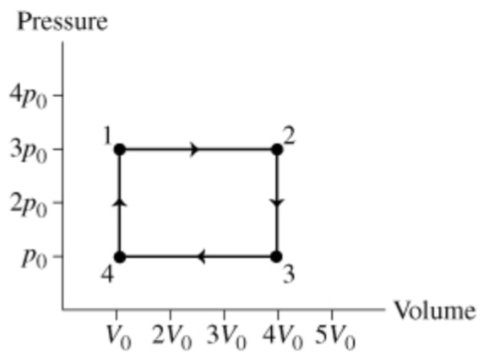# Problem: A) Find w12, the work done on the gas as it expands from state 1 to state 2.Express the work done in terms of Po and VoB) Find W23 , the work done on the gas as it cools from state 2 to state 3.Express your answer in terms of Po and Vo.C) Find W34, the work done on the gas as it is compressed from state 3 to state 4.Express your answer in terms of Po and Vo.D) Find W41, the work done on the gas as it is heated from state 4 to state 1.Express your answer in terms of Po and Vo .E)What is Wnet, the total work done on the gas during one cycle?Express your answer in terms of Po and Vo .F) When the gas is in state 1, its temperature is T1. Find the temperature T3 of the gas when it is in state 3. (Keep in mind that this is an ideal gas.)Express T3 in terms of T1.The diagram shows the pressure and volume of an ideal gas during one cycle of an engine. As the gas proceeds from state 1 to state 2, it is heated at constant pressure. It is then cooled at constant volume, until it reaches state 3. The gas is then cooled at constant pressure to state 4. Finally, the gas is heated at constant volume until it returns to state 1.

###### FREE Expert Solution

Work:

$\overline{){\mathbf{W}}{\mathbf{=}}{\mathbf{P}}{\mathbf{∆}}{\mathbf{V}}{\mathbf{=}}{\mathbf{n}}{\mathbf{R}}{\mathbf{T}}}$

A)

The work, W12 = PΔV

W12 = 3P0(4V0 - V0) = 9 P0V0

94% (191 ratings)###### Problem Details

A) Find w12, the work done on the gas as it expands from state 1 to state 2.
Express the work done in terms of Po and Vo

B) Find W23 , the work done on the gas as it cools from state 2 to state 3.

C) Find W34, the work done on the gas as it is compressed from state 3 to state 4.

D) Find W41, the work done on the gas as it is heated from state 4 to state 1.

E)What is Wnet, the total work done on the gas during one cycle?

F) When the gas is in state 1, its temperature is T1. Find the temperature T3 of the gas when it is in state 3. (Keep in mind that this is an ideal gas.)
Express T3 in terms of T1.

The diagram shows the pressure and volume of an ideal gas during one cycle of an engine. As the gas proceeds from state 1 to state 2, it is heated at constant pressure. It is then cooled at constant volume, until it reaches state 3. The gas is then cooled at constant pressure to state 4. Finally, the gas is heated at constant volume until it returns to state 1.